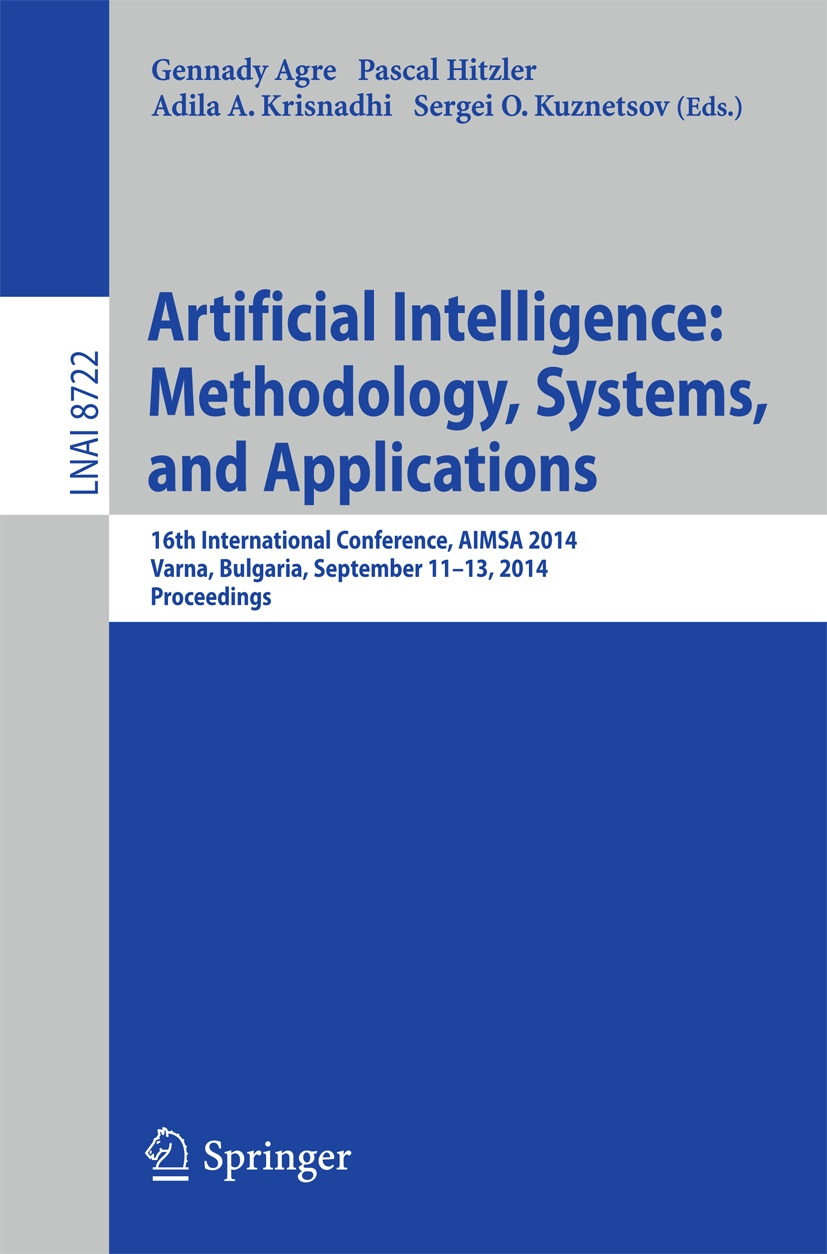• A
• A
• A
• ABC
• ABC
• ABC
• А
• А
• А
• А
• А
Regular version of the site

## Finetuning Randomized Heuristic Search for 2D Path Planning: Finding the Best Input Parameters for R* Algorithm through Series of Experiments

P. 278-285.
Yakovlev K., Baskin E., Hramolin I.

Path planning is typically considered in Artificial Intelligence as a graph searching problem and R* is state-of-the-art algorithm tailored to solve it. The algorithm decomposes given path finding task into the series of subtasks each of which can be easily (in computational sense) solved by well-known methods (such as A*). Parameterized random choice is used to perform the decomposition and as a result R* performance largely depends on the choice of its input parameters. In our work we formulate a range of assumptions concerning possible upper and lower bounds of R* parameters, their interdependency and their influence on R* performance. Then we evaluate these assumptions by running a large number of experiments. As a result we formulate a set of heuristic rules which can be used to initialize the values of R* parameters in a way that leads to algorithm’s best performance.

### In bookEdited by: G. Agre, P. Hitzler, A. A. Krisnadhi et al. Vol. 8722. Cham; Heidelberg; NY; Dordrecht; L.: Springer, 2014.